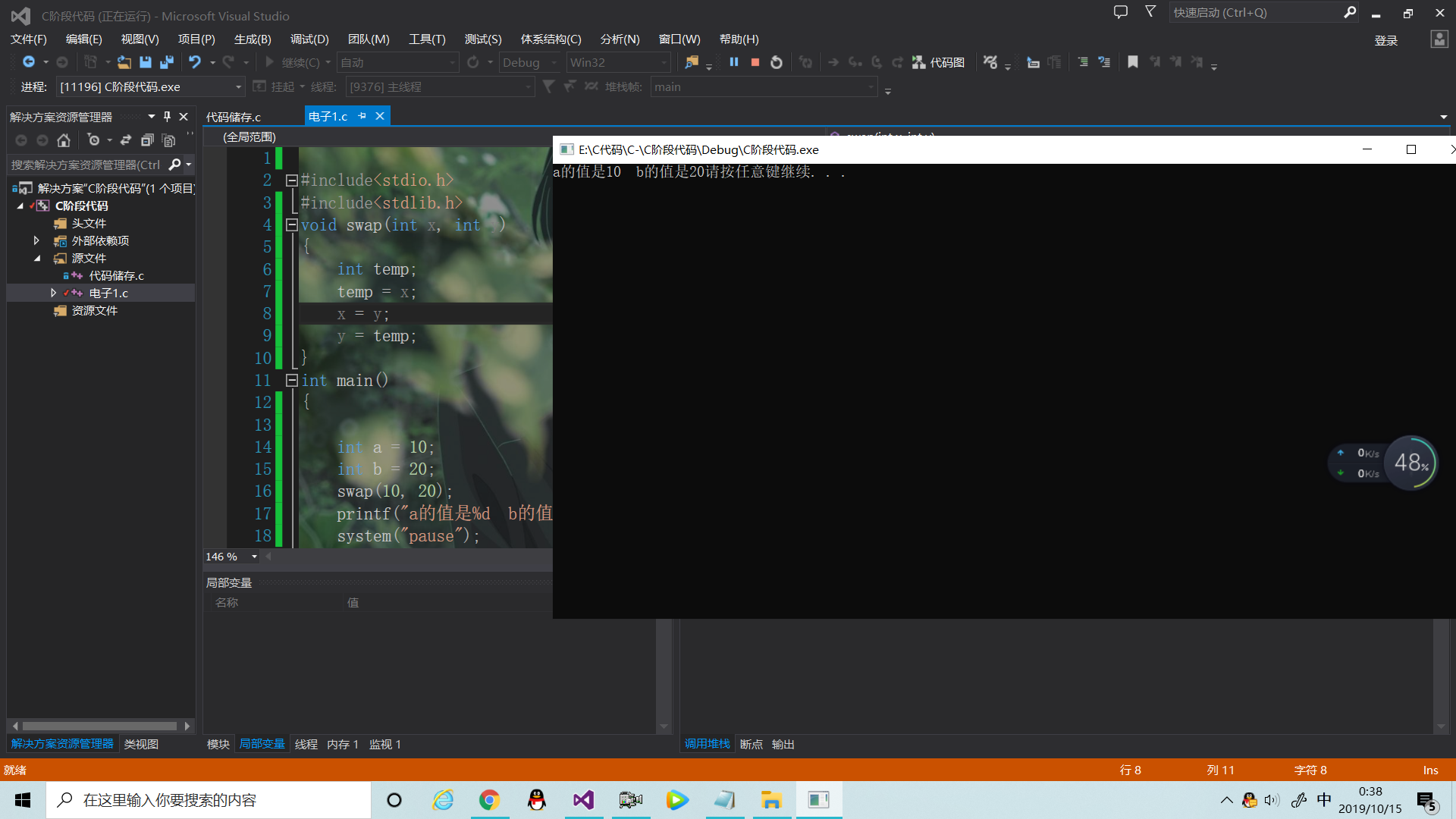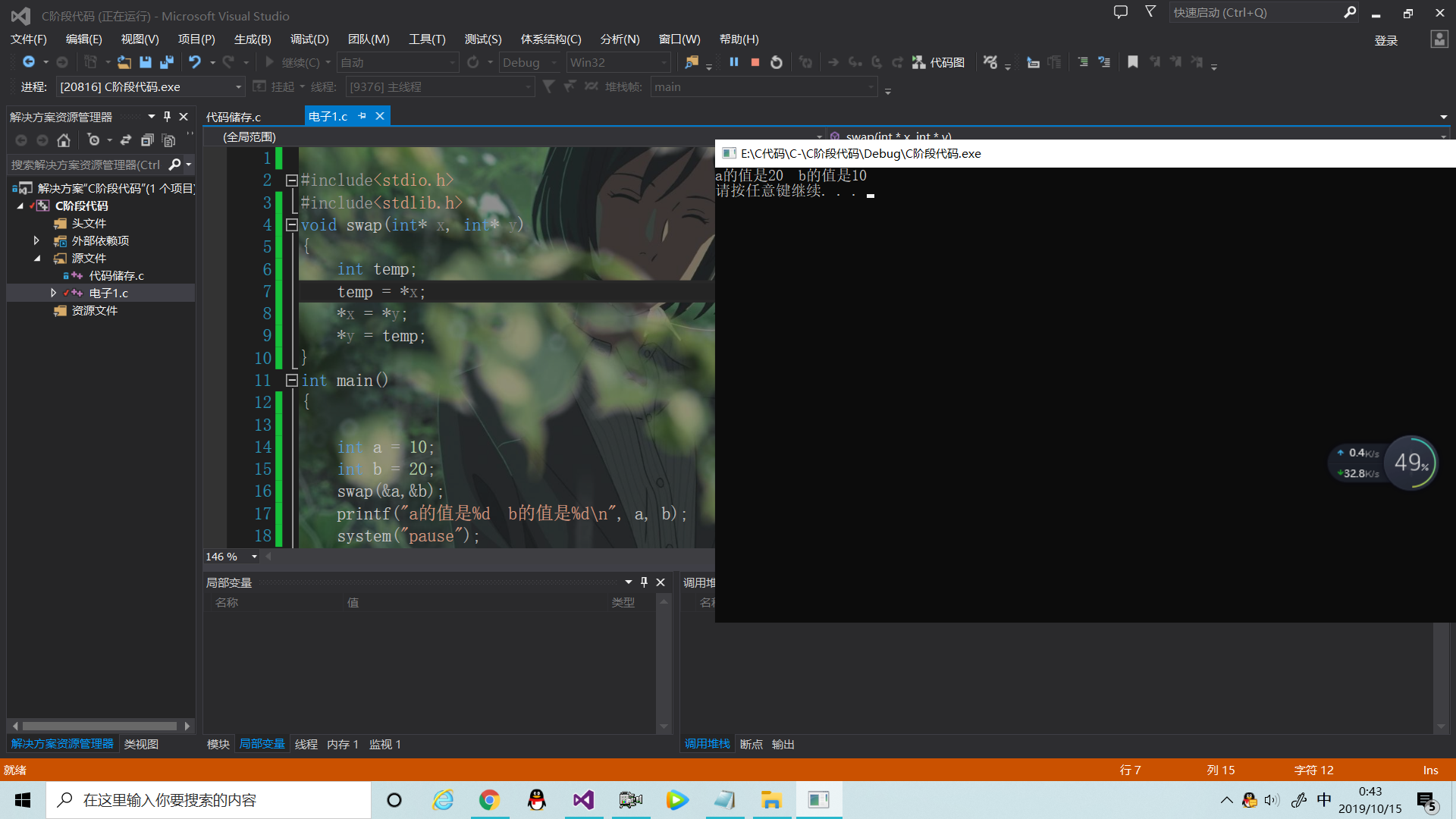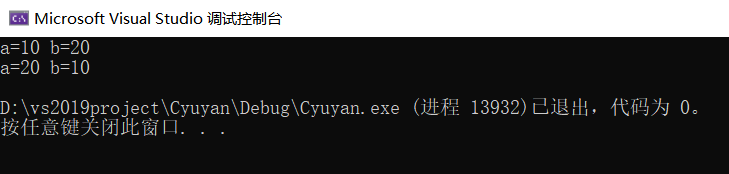• c++来交换两个数（指针交换法、引用交换法）
2022-04-04 15:44:11

### 交换两个数是任何语言的最基础的问题

本文就交换两个数提出他、两种不同的方法：

1.指针法交换两个数：

/*用指针的交换两个数*/

#include<iostream>
using namespace std;
void exchange(int *x , int *y)
{
int temp;
temp = *x;
*x = *y;
*y = temp;
}
int main()
{
int x = 10, y = 20;
cout <<"交换前：x ="<< x <<",y =" << y <<endl;
exchange(&x , &y);
cout <<" 交换后：x = "<< x <<",y ="<< y <<endl;
return 0;

}



解释：在这个方法中：在调用函数exchange时 *x 和 *y 实际上是在操作main函数中的x 和 y

由此来改变两个数值的大小

2.引用交换法：


/*通过引用来转换两个数*/
#include<iostream>
using namespace std;
void exchange(int &x , int &y)
{
int temp;
temp = x;
x = y;
y = temp;
}
int main()
{
int x  , y;
cout <<"请输入x和y的值：" <<endl;
cin >> x >> y;
cout << "交换前的x为：" << x <<",y 为："<< y <<endl;
exchange(x , y);
cout << "交换后的x为：" << x <<",y为："<< y << endl;
return 0;

}


解释：对于引用本质上就是为变量起一个小名，所以在exchange函数中int&x 和int &y在交换时就是在交换main函数中的x，y

感谢大家支持！c++
更多相关内容
• 两个数的进行交换 首先错误交换姿势: 因为形参是实参的副本,所以对形参这个"假身"进行操作 实参的是不会改变的 #include<stdio.h> #include<stdlib.h> void swap(int x, int y) { int temp; temp ...

两个数的值进行交换
首先错误交换姿势:
因为形参是实参的副本(拷贝),它们只是值相同,
形参和实参都有各自的地址,所以对形参这个"假身"进行操作
实参的值是不会改变的

#include<stdio.h>
#include<stdlib.h>
void swap(int x, int y)
{
int temp;
temp = x;
x = y;
y = temp;
}
int main()
{

int a = 10;
int b = 20;
swap(10, 20);
printf("a的值是%d  b的值是%d", a, b);
system("pause");
}


结果正确交换姿势
直接利用实参传递的地址对实参进行赋值操作
*x(解引用):根据房间号(内存地址),获取房间(内存地址)中的数据内容

#include<stdio.h>
#include<stdlib.h>
void swap(int* x, int* y)//定义了两个指针类型的参数
{			     //指针x,y分别对应的值是a,b的地址
int temp;
temp = *x;// 将a地址里面存储的值赋给temp
*x = *y;//将b地址里面的数据内容复制给a地址中的数据内容
*y = temp;//将temp的值赋给b (内存)地址中的数据内容
}
int main()
{

int a = 10;
int b = 20;
swap(&a,&b);//a,b将地址分别传递给指针x,y
printf("a的值是%d  b的值是%d\n", a, b);
system("pause");
}


结果展开全文• 下面小编就为大家带来一篇Java 交换两个变量的数值实现方法。小编觉得挺不错的，现在就分享给大家，也给大家做个参考。一起跟随小编过来看看吧
• //void Swap1(int x,int y)//并不能交换a b的 //{ // int tmp = 0; // tmp = x; // x = y; // y = tmp; //} //int main() //{ // int a = 10; // int b = 20; // int tmp = 0; // Swap1(a, b); // printf("a=%d b=...
#define _CRT_SECURE_NO_WARNINGS
#include <stdio.h>
//void Swap1(int x,int y)//并不能交换a b的值
//{
//	int tmp = 0;
//	tmp = x;
//	x = y;
//	y = tmp;
//}
//int main()
//{
//	int a = 10;
//	int b = 20;
//	int tmp = 0;
//	Swap1(a, b);
//	printf("a=%d b=%d\n", a,b);
//	return 0;
//}
void Swap2(int* x,int* y)//x y为指针变量
{
int tmp = 0;
tmp= *x;//将外面的a给tmp
*x= *y;//将外面的b给a
*y = tmp;//*在这里是解引用操作，例如*x:通过x存储的地址找到它所对应的内容，即a
}
int main()
{
int a = 10;
int b = 20;
printf("a=%d b=%d\n", a, b);
Swap2(&a, &b);
printf("a=%d b=%d\n", a, b);
return 0;
}
//int main()
//{
//	int a = 10;
//	int* p = &a;
//	printf("%p\n", &a); // 00EFFCB8 a的地址
//	printf("%p\n", p);  // 00EFFCB8 指针变量的值，指的是p本身的值，在这里是a的地址
//	printf("%p\n", &p); // 00EFFCAC 指针地址的值，指的是p的地址值，写作&p
//	return 0;
//}


运行结果：展开全文c语言
• 最近写代码时遇到一个问题，使用指针变量作为函数参数时，如何交换两个数的。这个问题看似简单，但却关系到对C语言中指针的理解程度。请看下面三个swap函数#include "stdafx.h"void swap1(int a,int b){int t;t=a;...

最近写代码时遇到一个问题，使用指针变量作为函数参数时，如何交换两个数的值。这个问题看似简单，但却关系到对C语言中指针的理解程度。请看下面三个swap函数

#include "stdafx.h"

void swap1(int a,int b)

{

int t;

t=a;a=b;b=t;

printf("a:%d,b:%d\n",a,b);

}

void swap2(int *a,int *b)

{

int *t;

t=a;a=b;b=t;

}

void swap3(int *a,int *b)

{

int t;

t=*a;*a=*b;*b=t;

}

int main(int argc, char* argv[])

{

int a =5,b=9;

swap1(a,b);

printf("a:%d,b:%d\n",a,b);

swap2(&a,&b);

printf("a:%d,b:%d\n",a,b);

swap3(&a,&b);

printf("a:%d,b:%d\n",a,b);

return 0;

}

第一个swap，定义为void swap1(int a,int b)，如果使用java或者其它高级语言，使用该函数没什么问题，甚至是天经地义的，但是C语言里却不行，原因是main中调用swap1()时，将实参a，b的值传递给了交换函数，如果此时在swap1中打印，可以看到a为9，b为5，但是，由于未改变a,b所对应的地址的值，当函数退出时，形参a，b的值已经释放，地址的值未发生改变，因此这里不会返回任何结果，。因此main()中第一行打印的结果仍然未a:5,b:9。

第二个swap，看似的确使用了指针，但输出的也是“a:5,b:9”，为什么呢？由于未给t赋值，t中并没有确定的值，索引t中的值是不可预见的。此时t可能指向一个未知的存储单元，而严重的情况是，该单元的数据可能是有用的，因此swap2()不但没有实现两个数的交换，反而给系统的稳定性带来威胁。本例的作用是将t作为临时指针变量，实现&a和&b的地址交换，而值并未发生变化。

第三个swap是正确的。为了在函数中改变了的变量能被其它函数调用，正确的办法是用指针变量作为函数参数，在函数执行过程中使指针变量所指向的变量值发生变化，函数调用结束后，哲别变量值的变化依然保留下来，这样就实现了通过函数调用是变量的值发生变化，在其它函数中可以使用这些改变了的值的目的。

展开全文• 关于用指针交换两个数 西邮Linux 兴趣小组又到了纳新季，作为一个老学长，面试学弟学妹们的时候，必然要然他们感受到小组的关怀(受尽折磨)，这一届孩子还是挺强的，但是一到指针上就栽了坑，在加上我们小组的陈年老...
• a,b的不会改变，因为在子函数Swap中a和b的数值进行了交换，但是子函数数据中的改变不会影响到父函数 void Swap_error1(int a,int b) { int tmp=a; a=b; b=tmp; } int main() { int a=10; int b=20; Swap_...c语言
• //交换两个数的函数实现的原型 int main(void) { int a, b; printf(“请输入你要交换的整数:\n”); scanf_s("%d%d", &a, &b); swap(&a, &b); printf(“交换后的数为%d %d”, a, b); syc语言 开发语言
• //交换两个数的函数实现的原型 int main(void) { int a, b; printf(“请输入两个整数\n”); scanf_s("%d", &a);//将两个整数读入到缓存区中去 scanf_s("%d", &b); change(&a, &b);//为c语言
• 《程序设计基础实训指导教程-c语言》 ...【实训内容1】使用指针交换两个数 #include <stdio.h> int main() { int a,b,*p; int t; p=&t; scanf("%d %d",&a,&b); *p=a; a=...c语言
• 其实这题是要结构把学生成绩输进去再根据程序排序再输出学生信息，我不知道怎么根据成绩大小把st[].name输出啊，我了一个交换函数，change想交换st[j].name和st[j-1].name但是都不行，因为st[j].name是常量...
• //用指针交换两个值的内容 #define _CRT_SECURE_NO_WARNINGS #include <stdio.h> #include <stdlib.h> void swap(int* x, int* y); void swap(int* x, int* y) { int t; t = *x; ...c语言
• void Swap_err1(int a, int b)//error，形参交换，实参没有交换 { int tmp = a; a = b; b = tmp; } void Swap_err2(int* p1, int* p2)//error,没有解引用 { int* tmp; tmp = p1; p1 = p2; p2 = tmp; } void ...c语言
• 定义一个函数Swap，功能为交换两个变量的。 不用指针直接交换（错误）。 例： #include<stdio.h> void Swap_err(int a,int b) { int tmp = a; a = b; b = tmp; } int main() { int a=10; int b=20; ...
• #include<stdio.h> void main() { int *p1 , *p2 , p; //这里的 “ ” 代表定义 int a,b; scanf("%d %d" , &a,&b); p1=&a; //a的地址给了p1 ... //进行地址的交换 p1=p2...
• 交换两个变量的，也就是交换两块内存区域中储存的。 而通过指针，能够对该指针指向对内存区域进行读写。 代码 //定义交换函数，形参为两个指向变量地址指针 void Swap(int * p1, int * p2){ //p1,p2是指针，...c语言
• 使用外部函数交换两个变量的，一个再简单不过的事情，但是在C/C++中，正确实现该功能反应了你对指针和引用等重要知识的掌握程度。本文列举了几种常见的写法，其中前三种是错误的，后两种是正确的。第四种使用的是...
• 使用指针参数交换两个变量”案例C主讲教师崔玲玲 5.1 “【案例说明】函数实现两个变量的交换使其在主调函数和被调函数中的一致。要求用指针变量作为函数参数。程序运行结果如图 5.1所示。图 5.1 使用指针...
• #include&lt;cstdio&gt; int a,b,t; int *p, *q; int main() { scanf("...//交换两个指针所指向的元素 *p = *q; *q = t; printf("%d %d",a,b); return 0; } ...
• 转载于:https://www.cnblogs.com/lenglangjin/p/6601950.html
• 用户自定义函数使用指针交换两个变量 将指针变量的赋值给变量，然后将b指针变量的赋值给a指针变量，最后之前存储a指针变量的ch赋值给b指针变量，完成两个指针变量的地址交换，最后在main函数中调用...c语言
• 问题：请使用指针变量自定义函数，实现两个整数交换 #include <stdio.h> int fun1(int *a , int *b); void init(); int main() { init(); return 0; } int fun1(int *a , int *b) { *a = *a+*b; *b = ...c语言 开发语言 后端
• 如何传址的方式交换两个数的大小 首先先看第一个程序，函数的调用来解决看似正确实则运行出来的结果是错误的 #define _CRT_SECURE_NO_WARNINGS 1 #include #include #include int JH(int x, int y); int main() ...c语言
• #include <stdio.h> #include <stdlib.h> int main() { void changeNum(int *u,int *v); printf("Hello world!\n"); int a=5,b=6; printf("a=%d,b=%d\n",a,b); if( a < b){...c语言
• 【问题描述】 编写函数int Read(int *... 交换主函数里的两个整形数组a和b里的数据，其中ab中数据元素的实际个数传递给形参m和n。 编写函数void Sort(int *x, int n); 负责实现数组元素从小到大的排序。 【样例输.排序算法 数据结构 c语言 c++
• C语言中交换两个变量的可以在主函数中直接实现，也可以通过子函数来实现。下面我们介绍子函数实现的情况。 1.将两个变量的作为实参直接传递，在子函数内部定义一个普通变量来对两个形参进行交换，并分别在子...C语言
•c语言
• #include <stdio.h> void main() { int a = 10; int b = 100; int *p = &a; int *q = &b; int t = *p; *p = *q; q = &t; printf("%d %d",*p,*q); }...